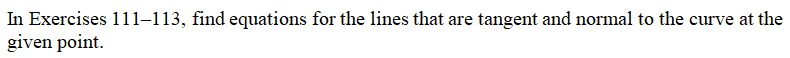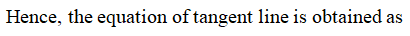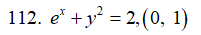# In Exercises 111–116, find equations for the lines that are tangent and normal to the curve at the given point.111. x2 + 2y2 = 9, (1, 2)112. ex + y2 = 2, (0, 1)113. xy + 2x - 5y = 2, (3, 2)114. (y - x)2 = 2x + 4, (6, 2)115. x + sqrt(xy) = 6, (4, 1)116. x^(3/2) + 2y^(3/2) = 17, (1, 4)

Question
2 views

In Exercises 111–116, find equations for the lines that are tangent and normal to the curve at the given point.

111. x2 + 2y2 = 9, (1, 2)

112. ex + y2 = 2, (0, 1)

113. xy + 2x - 5y = 2, (3, 2)

114. (y - x)2 = 2x + 4, (6, 2)

115. x + sqrt(xy) = 6, (4, 1)

116. x^(3/2) + 2y^(3/2) = 17, (1, 4)

check_circle

Step 1Step 2Step 3...

### Want to see the full answer?

See Solution

#### Want to see this answer and more?

Solutions are written by subject experts who are available 24/7. Questions are typically answered within 1 hour.*

See Solution
*Response times may vary by subject and question.
Tagged in

### Calculus